×
Log in to StudySoup
Get Full Access to Engineering Mechanics: Statics - 14 Edition - Chapter 4 - Problem 4-40
Join StudySoup for FREE
Get Full Access to Engineering Mechanics: Statics - 14 Edition - Chapter 4 - Problem 4-40

Already have an account? Login here
×
Reset your password

# Answer: The curved rod has a radius of 5 ft. If a force ofISBN: 9780133918922 126

## Solution for problem 4-40 Chapter 4

Engineering Mechanics: Statics | 14th Edition

• Textbook Solutions
• 2901 Step-by-step solutions solved by professors and subject experts
• Get 24/7 help from StudySoup virtual teaching assistantsEngineering Mechanics: Statics | 14th Edition

4 5 1 365 Reviews
26
3
Problem 4-40

The curved rod has a radius of 5 ft. If a force of 60 lb acts at its end as shown, determine the moment of this force about point C.

Step-by-Step Solution:
Step 1 of 3

Problem 4-40

The curved rod has a radius of 5 ft. If a force of 60 lb acts at its end as shown, determine the moment of this force about point C.

Step-by-Step Solution

Step  1 of 3

First define vectors: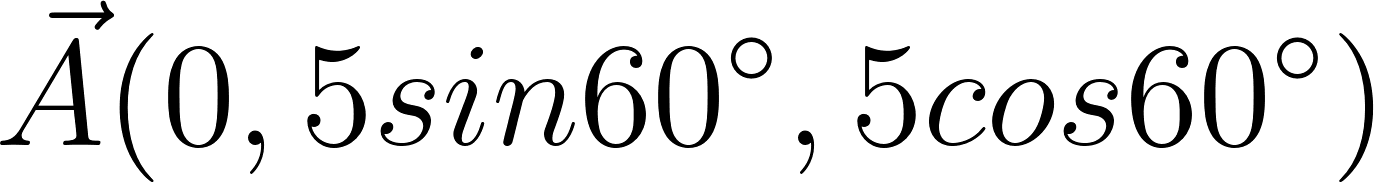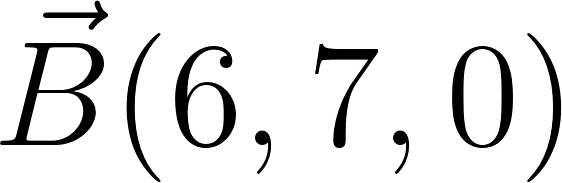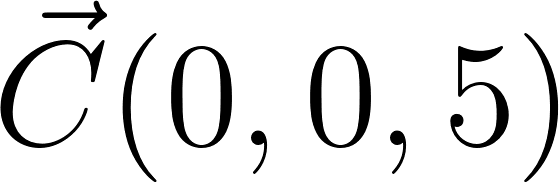Step  2 of 3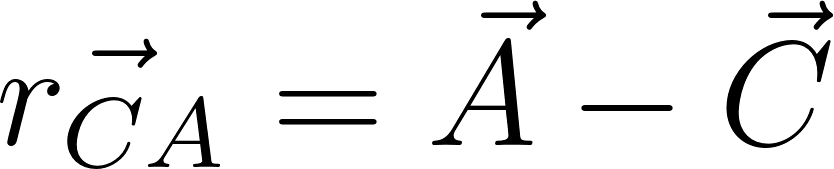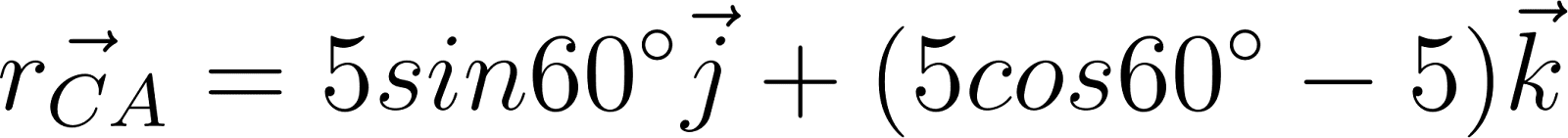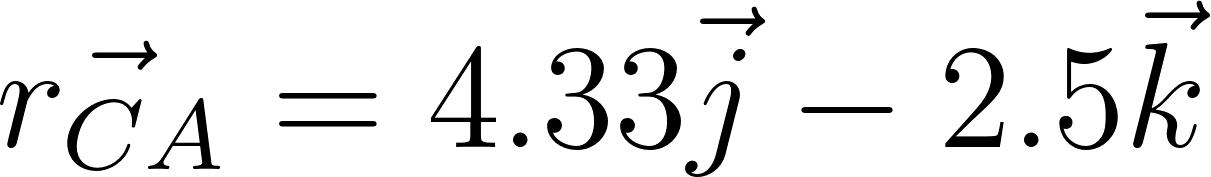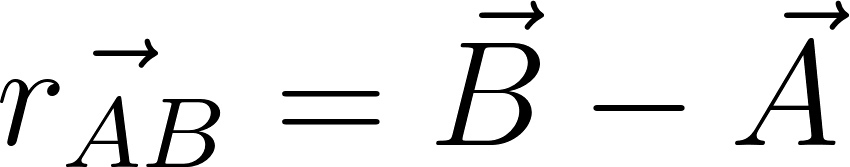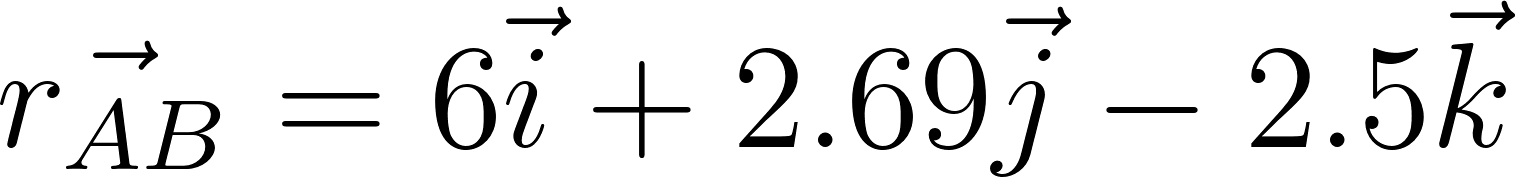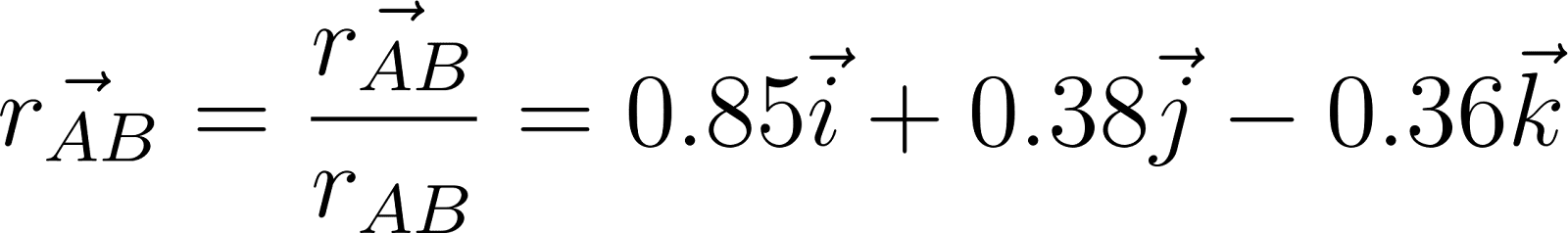Step  3 of 3Now do vector multiplication of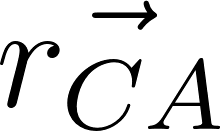and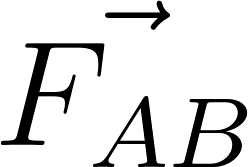Moment about C is,Step 2 of 3

Step 3 of 3

##### ISBN: 9780133918922

The answer to “The curved rod has a radius of 5 ft. If a force of 60 lb acts at its end as shown, determine the moment of this force about point C.” is broken down into a number of easy to follow steps, and 30 words. Since the solution to 4-40 from 4 chapter was answered, more than 380 students have viewed the full step-by-step answer. The full step-by-step solution to problem: 4-40 from chapter: 4 was answered by , our top Engineering and Tech solution expert on 11/10/17, 05:25PM. Engineering Mechanics: Statics was written by and is associated to the ISBN: 9780133918922. This textbook survival guide was created for the textbook: Engineering Mechanics: Statics, edition: 14. This full solution covers the following key subjects: Force, its, determine, end, acts. This expansive textbook survival guide covers 11 chapters, and 1136 solutions.

Unlock Textbook Solution

Enter your email below to unlock your verified solution to:

Answer: The curved rod has a radius of 5 ft. If a force of